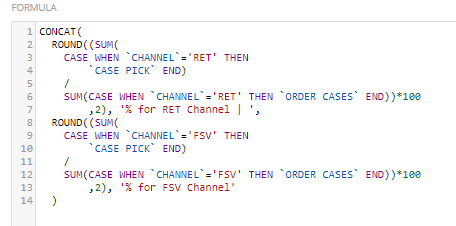# Average if channel type statement beast mode

Hello,

I am trying to create a summary number that takes an average of one customer type and compares it to another.

My code currently looks like

CONCAT(
(CASE when `Channel` = 'RET' then SUM(`ordercases`)),' | '
(CASE when `Channel` = 'FSV' then SUM(`ordercases`))

I am having trouble with the syntax. Has anyone had any experience with this?

Thanks much!

Update I figured out the code to get it to work

CONCAT(

(SUM(CASE when `Channel`='RET' then `ordercases`end)),' | ',

(SUM(CASE when `Channel`='FSV' then `ordercases`end))
)

Would it be possible to put a custom calculation at the beginning and get it to work too?

The equation I would like to put in front would be sum(case pick)/ sum(order cases) so basically getting an overall average

• I think that something like this would work as a summary number for this then:(I think it is easier to read in the photo, but I will paste the text here as well)  good luck!

CONCAT(
ROUND((SUM(
CASE WHEN `CHANNEL`='RET' THEN
`CASE PICK` END)
/
SUM(CASE WHEN `CHANNEL`='RET' THEN `ORDER CASES` END))*100
,2), '% for RET Channel | ',
ROUND((SUM(
CASE WHEN `CHANNEL`='FSV' THEN
`CASE PICK` END)
/
SUM(CASE WHEN `CHANNEL`='FSV' THEN `ORDER CASES` END))*100
,2), '% for FSV Channel'
)

______________________________________________________________________________________________
“There is a superhero in all of us, we just need the courage to put on the cape.” -Superman
______________________________________________________________________________________________

• Is anyone able to help out with this request?

• Hello @KVincent

You should be able to add this calculation into your previous beastmode.
If you just replace the word "newcalc." with your new calculation it should show in your summary number.

CONCAT( (newcalc.),

(SUM(CASE when `Channel`='RET' then `ordercases`end)),' | ',

(SUM(CASE when `Channel`='FSV' then `ordercases`end))
)

**Say “Thanks" by clicking the thumbs up in the post that helped you.
**Please mark the post that solves your problem by clicking on "Accept as Solution"
• You should be able to add as many calculations to that con at statement as you want.

CONCAT(

ROUND((SUM(case pick calculation) / SUM(order cases calculation))*100,2), ‘% | ‘,
(SUM(CASE when `Channel`='RET' then `ordercases`end)),' | ',

(SUM(CASE when `Channel`='FSV' then `ordercases`end))
)

i am am not sure what your calculations are for case pick and order cases. But this will give you the percent rounded to 2 decimals.

______________________________________________________________________________________________
“There is a superhero in all of us, we just need the courage to put on the cape.” -Superman
______________________________________________________________________________________________
• Thanks for your reply. So case pick and order cases are individual fields and I am trying to get the average of each othem in beast mode calculation. So basically it would look like this.

CONCAT(

ROUND((SUM(case pick calculation) / SUM(order cases calculation))**when channel = 'RET')**100,2), '% | ', ROUND((SUM(case pick calculation) / SUM(order cases calculation))**when channel = 'FSV')**100,2), ‘% ‘

End)

Would this be possible? Not sure how the string would look. Thanks you so much for your time.

• Thats perfect thank you so much! I have been pondering over this one for weeks now!